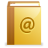Contact Us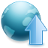Upload eBook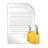Privacy Policy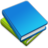New eBooks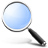Search Engine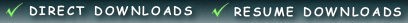# MATLAB Differential and Integral Calculus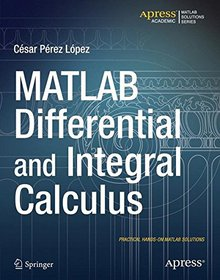### Book Details:

 Publisher: Apress Series: Apress Author: Cesar Lopez Edition: 1 ISBN-10: 1484203054 ISBN-13: 9781484203057
 Pages: 228 Published: Oct 02 2014 Posted: Apr 16 2016 Language: English Book format: PDF Book size: 5.03 MB

### Book Description:

MATLAB is a high-level language and environment for numerical computation, visualization, and programming. Using MATLAB, you can analyze data, develop algorithms, and create models and applications. The language, tools, and built-in math functions enable you to explore multiple approaches and reach a solution faster than with spreadsheets or traditional programming languages, such as C/C++ or Java. MATLAB Differential and Integral Calculus introduces you to the MATLAB language with practical hands-on instructions and results, allowing you to quickly achieve your goals. In addition to giving a short introduction to the MATLAB environment and MATLAB programming, this book provides all the material needed to work with ease in differential and integral calculus in one and several variables. Among other core topics of calculus, you will use MATLAB to investigate convergence, find limits of sequences and series and, for the purpose of exploring continuity, limits of functions. Various kinds of local approximations of functions are introduced, including Taylor and Laurent series. Symbolic and numerical techniques of differentiation and integration are covered with numerous examples, including applications to finding maxima and minima, areas, arc lengths, surface areas and volumes. You will also see how MATLAB can be used to solve problems in vector calculus and how to solve differential and difference equations.

### Related Books:

#### MATLAB Differential Equations

MATLAB is a high-level language and environment for numerical computation, visualization, and programming. Using MATLAB, you can analyze data, develop algorithms, and create models and applications. The language, tools, and built-in math functions enable you to explore multiple approaches and reach a solution faster than with spreadsheets or traditional programming languages, such as C/C++ or Java.MATLAB Differential Equations introduces you to the MATLAB language with practical hands-on instructions and results, allowing you to quickly achieve your goals. In addition to giving an introduction to the MATLAB environment and MATLAB programming, this book provides all the material needed to work on differential equations using MATLAB. It includes techni...

#### Signals and Systems

##### With MATLAB Computing and Simulink Modeling
4th Edition
This text contains a comprehensive discussion on continuous and discrete time signals and systems with many MATLAB examples. It is written for junior and senior electrical and computer engineering students, and for self-study by working professionals. The prerequisites are a basic course in differential and integral calculus, and basic electric circuit theory. To get the most out of this text, it is highly recommended that Appendix A is thoroughly reviewed. This appendix serves as an introduction to MATLAB, and is intended for those who are not familiar with it. The Student Edition of MATLAB is an inexpensive, and yet a very powerful software package. ...

#### Signals and Systems

##### With MATLAB Computing and Simulink Modeling
3rd Edition
This text has been superseded by the FOURTH EDITION which includes a comprehensive discussion on window functions and examples using the fft and ifft MATLAB functions. As in previous editions, this text is an introduction to continuous and discrete-time signals and systems. It contains numerous examples that are solved analytically and verified with the latest Student Versions of MATLAB. It is intended for electrical engineering majors. It can also be used for self-study. Freshman-level calculus and some elementary knowledge of differential equations is assumed. An introduction to MATLAB, Simulink, review of complex algebra, and matrices and determinan...